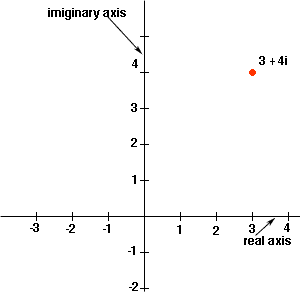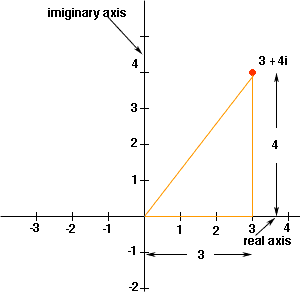Quandaries and Queries hello... how would you do a question like |3+4i|?  is that different than just doing 3+4i?    i'm a student in 10th grade. thank you, sandy Hi Sandy, I am not exactly sure what you are asking. What I am going to explain is the difference between 3+4i and |3+4i|. There is both an algebraic/numeric and a geometric interpretation of these quantities. Algebraic/Numeric: 3 + 4i is a complex number with real part 3 and imiginary part 4. The quantity |3+4i| is a real number defined by the square root of 32+42. Thus |3+4i| = root(32+42) = root(25) = 5 Geometric: You can plot complex numbers in a two-dimensional diagram by making the horizontal axis the real part of the number and the vertical axis the imiginary part of the number.The quantity |3+4i| is then the distance from the origin to 3+4i. From the diagram you can see that this distance can be calculated using Pythagoras' Theorem.Thus, again |3+4i| = root(32+42) = root(25) = 5 Penny Go to Math Central